# electomagnetics 2

Permeability

The nonlinearity of material permeability may be graphed for better understanding. We’ll place the quantity of field intensity (H), equal to field force (mmf) divided by the length of the material, on the horizontal axis of the graph. On the vertical axis, we’ll place the quantity of flux density (B), equal to total flux divided by the cross-sectional area of the material. We will use the quantities of field intensity (H) and flux density (B) instead of field force (mmf) and total flux (Φ) so that the shape of our graph remains independent of the physical dimensions of our test material. What we’re trying to do here is show a mathematical relationship between field force and flux for any chunk of a particular substance, in the same spirit as describing a material’s specific resistance in ohm-cmil/ft instead of its actual resistance in ohms.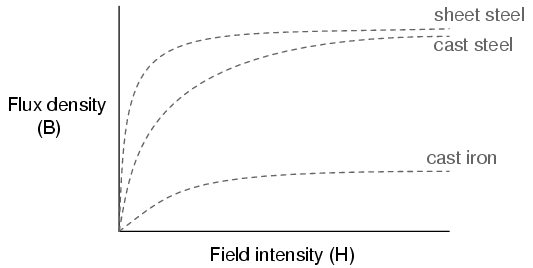This is called the normal magnetization curve, or B-H curve, for any particular material. Notice how the flux density for any of the above materials (cast iron, cast steel, and sheet steel) levels off with increasing amounts of field intensity. This effect is known as saturation. When there is little applied magnetic force (low H), only a few atoms are in alignment, and the rest are easily aligned with additional force. However, as more flux gets crammed into the same cross-sectional area of a ferromagnetic material, fewer atoms are available within that material to align their electrons with additional force, and so it takes more and more force (H) to get less and less “help” from the material in creating more flux density (B). To put this in economic terms, we’re seeing a case of diminishing returns (B) on our investment (H). Saturation is a phenomenon limited to iron-core electromagnets. Air-core electromagnets don’t saturate, but on the other hand they don’t produce nearly as much magnetic flux as a ferromagnetic core for the same number of wire turns and current.

Another quirk to confound our analysis of magnetic flux versus force is the phenomenon of magnetic hysteresis. As a general term, hysteresis means a lag between input and output in a system upon a change in direction. Anyone who’s ever driven an old automobile with “loose” steering knows what hysteresis is: to change from turning left to turning right (or vice versa), you have to rotate the steering wheel an additional amount to overcome the built-in “lag” in the mechanical linkage system between the steering wheel and the front wheels of the car. In a magnetic system, hysteresis is seen in a ferromagnetic material that tends to stay magnetized after an applied field force has been removed (see “retentivity” in the first section of this chapter), if the force is reversed in polarity.

Let’s use the same graph again, only extending the axes toindicate both positive and negative quantities. First we’ll apply an increasing field force (current through the coils of our electromagnet). We should see the flux density increase (go up and to the right) according to the normal magnetization curve:Next, we’ll stop the current going through the coil of the electromagnet and see what happens to the flux, leaving the first curve still on the graph: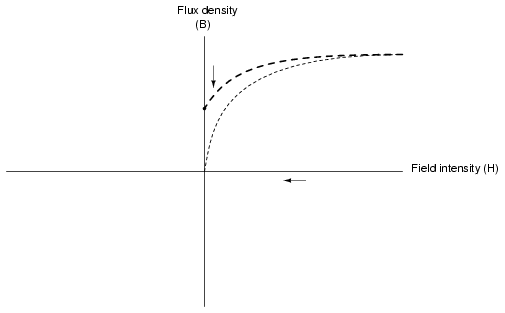Due to the retentivity of the material, we still have a magnetic flux with no applied force (no current through the coil). Our electromagnet core is acting as a permanent magnet at this point. Now we will slowly apply the same amount of magnetic field force in the opposite direction to our sample:The flux density has now reached a point equivalent to what it was with a full positive value of field intensity (H), except in the negative, or opposite, direction. Let’s stop the current going through the coil again and see how much flux remains:Once again, due to the natural retentivity of the material, it will hold a magnetic flux with no power applied to the coil, except this time its in a direction opposite to that of the last time we stopped current through the coil. If we re-apply power in a positive direction again, we should see the flux density reach its prior peak in the upper-right corner of the graph again:The “S”-shaped curve traced by these steps form what is called the hysteresis curve of a ferromagnetic material for a given set of field intensity extremes (-H and +H). If this doesn’t quite make sense, consider a hysteresis graph for the automobile steering scenario described earlier, one graph depicting a “tight” steering system and one depicting a “loose” system: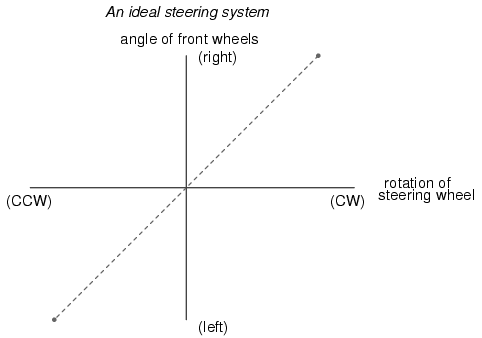Just as in the case of automobile steering systems, hysteresis can be a problem. If you’re designing a system to produce precise amounts of magnetic field flux for given amounts of current, hysteresis may hinder this design goal (due to the fact that the amount of flux density would depend on the current and how strongly it was magnetized before!). Similarly, a loose steering system is unacceptable in a race car, where precise, repeatable steering response is a necessity. Also, having to overcome prior magnetization in an electromagnet can be a waste of energy if the current used to energize the coil is alternating back and forth (AC). The area within the hysteresis curve gives a rough estimate of the amount of this wasted energy.

Other times, magnetic hysteresis is a desirable thing. Such is the case when magnetic materials are used as a means of storing information (computer disks, audio and video tapes). In these applications, it is desirable to be able to magnetize a speck of iron oxide (ferrite) and rely on that material’s retentivity to “remember” its last magnetized state. Another productive application for magnetic hysteresis is in filtering high-frequency electromagnetic “noise” (rapidly alternating surges of voltage) from signal wiring by running those wires through the middle of a ferrite ring. The energy consumed in overcoming the hysteresis of ferrite attenuates the strength of the “noise” signal. Interestingly enough, the hysteresis curve of ferrite is quite extreme: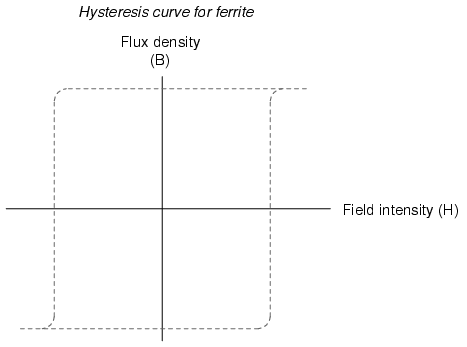• REVIEW:
• The permeability of a material changes with the amount of magnetic flux forced through it.
• The specific relationship of force to flux (field intensity H to flux density B) is graphed in a form called the normal magnetization curve.
• It is possible to apply so much magnetic field force to a ferromagnetic material that no more flux can be crammed into it. This condition is known as magnetic saturaton.
• When the retentivity of a ferromagnetic substance interferes with its re-magnetization in the opposite direction, a condition known as hysteresis occurs.

## Electromagnetic induction

While Oersted’s surprising discovery of electromagnetism paved the way for more practical applications of electricity, it was Michael Faraday who gave us the key to the practical generation of electricity: electromagnetic induction. Faraday discovered that a voltage would be generated across a length of wire if that wire was exposed to a perpendicular magnetic field flux of changing intensity.

An easy way to create a magnetic field of changing intensity is to move a permanent magnet next to a wire or coil of wire. Remember: the magnetic field must increase or decrease in intensity perpendicular to the wire (so that the lines of flux “cut across” the conductor), or else no voltage will be induced: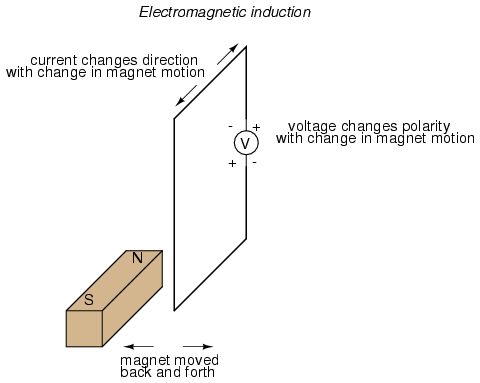Faraday was able to mathematically relate the rate of change of the magnetic field flux with induced voltage (note the use of a lower-case letter “e” for voltage. This refers to instantaneous voltage, or voltage at a specific point in time, rather than a steady, stable voltage.):The “d” terms are standard calculus notation, representing rate-of-change of flux over time. “N” stands for the number of turns, or wraps, in the wire coil (assuming that the wire is formed in the shape of a coil for maximum electromagnetic efficiency).

This phenomenon is put into obvious practical use in the construction of electrical generators, which use mechanical power to move a magnetic field past coils of wire to generate voltage. However, this is by no means the only practical use for this principle.

If we recall that the magnetic field produced by a current-carrying wire was always perpendicular to that wire, and that the flux intensity of that magnetic field varied with the amount of current through it, we can see that a wire is capable of inducing a voltage along its own length simply due to a change in current through it. This effect is called self-induction: a changing magnetic field produced by changes in current through a wire inducing voltage along the length of that same wire. If the magnetic field flux is enhanced by bending the wire into the shape of a coil, and/or wrapping that coil around a material of high permeability, this effect of self-induced voltage will be more intense. A device constructed to take advantage of this effect is called an inductor, and will be discussed in greater detail in the next chapter.

• REVIEW:
• A magnetic field of changing intensity perpendicular to a wire will induce a voltage along the length of that wire. The amount of voltage induced depends on the rate of change of the magnetic field flux and the number of turns of wire (if coiled) exposed to the change in flux.
• Faraday’s equation for induced voltage: e = N(dΦ/dt)
• A current-carrying wire will experience an induced voltage along its length if the current changes (thus changing the magnetic field flux perpendicular to the wire, thus inducing voltage according to Faraday’s formula). A device built specifically to take advantage of this effect is called an inductor.

## Mutual inductance

If two coils of wire are brought into close proximity with each other so the magnetic field from one links with the other, a voltage will be generated in the second coil as a result. This is called mutual inductance: when voltage impressed upon one coil induces a voltage in another.

A device specifically designed to produce the effect of mutual inductance between two or more coils is called a transformer.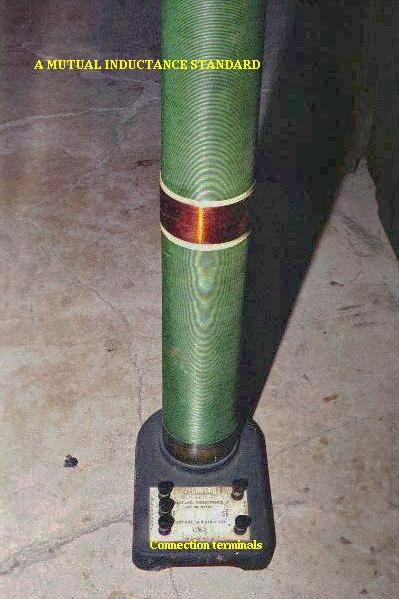width=”399″ height=”599″/>

The device shown in te above photograph is a kind of transformer, with two concentric wire coils. It is actually intended as a precision standard unit for mutual inductance, but for the purposes of illustrating what the essence of a transformer is, it will suffice. The two wire coils can be distinguished from each other by color: the bulk of the tube’s length is wrapped in green-insulated wire (the first coil) while the second coil (wire with bronze-colored insulation) stands in the middle of the tube’s length. The wire ends run down to connection terminals at the bottom of the unit. Most transformer units are not built with their wire coils exposed like this.

Because magnetically-induced voltage only happens when the magnetic field flux is changing in strength relative to the wire, mutual inductance between two coils can only happen with alternating (changing — AC) voltage, and not with direct (steady — DC) voltage. The only applications for mutual inductance in a DC system is where some means is available to switch power on and off to the coil (thus creating a pulsing DC voltage), the induced voltage peaking at every pulse.

A very useful property of transformers is the ability to transform voltage and current levels according to a simple ratio, determined by the ratio of input and output coil turns. If the energized coil of a transformer is energized by an AC voltage, the amount of AC voltage induced in the unpowered coil will be equal to the input voltage multiplied by the ratio of output to input wire turns in the coils. Conversely, the current through the windings of the output coil compared to the input coil will follow the opposite ratio: if the voltage is increased from input coil to output coil, the current will be decreased by the same proportion. This action of the transformer is analogous to that of mechanical gear, belt sheave, or chain sprocket ratios:A transformer designed to output more voltage than it takes in across the input coil is called a “step-up” transformer, while one designed to do the opposite is called a “step-down,” in reference to the transformation of voltage that takes place. The current through each respective coil, of course, follows the exact opposite proportion.

• REVIEW:
• Mutual inductance is where the magnetic field generated by a coil of wire induces voltage in an adjacent coil of wire.
• A transformer is a device constructed of two or more coils in close proximity to each other, with the express purpose of creating a condition of mutual inductance between the coils.
• Transformers only work with changing voltages, not steady voltages. Thus, they may be classified as an AC device and not a DC device.

Related

COMMENT songs